# Factors

An introduction to working categorial variables using factors in R
module 5
week 7
tidyverse
factors
categorial variables
Author
Affiliation

Department of Biostatistics, Johns Hopkins

Published

October 11, 2022

# Pre-lecture materials

Before class, you can prepare by reading the following materials:

1. Wrangling Categorical Data in R by Amelia McNamara, Nicholas J Horton
2. https://swcarpentry.github.io/r-novice-inflammation/12-supp-factors
3. https://forcats.tidyverse.org

### Acknowledgements

Material for this lecture was borrowed and adopted from

# Learning objectives

Learning objectives

At the end of this lesson you will:

• How to create factors and some challenges working with them in base R
• An introduction to the `forcats` package in the `tidyverse` to work with categorical variables in R

# Introduction

Factors are used for working with categorical variables, or variables that have a fixed and known set of possible values (income bracket, U.S. state, political affiliation).

Factors are useful when:

• You want to include categorical variables in regression models
• You want to plot categorical data (e.g. want to map categorical variables to aesthetic attributes)
• You want to display character vectors in a non-alphabetical order
Example

Imagine that you have a variable that records month:

``x <- c("Dec", "Apr", "Jan", "Mar")``

Using a string to record this variable has two problems:

1. There are only twelve possible months, and there’s nothing saving you from typos:
``x_typo <- c("Dec", "Apr", "Jam", "Mar")``
1. It doesn’t sort in a useful way:
``sort(x)``
`` "Apr" "Dec" "Jan" "Mar"``

## Factor basics

You can fix both of these problems with a factor.

To create a factor you must start by creating a list of the valid levels:

``````month_levels <- c(
"Jan", "Feb", "Mar", "Apr", "May", "Jun",
"Jul", "Aug", "Sep", "Oct", "Nov", "Dec"
)``````

Now we can create a factor with the `factor()` function defining the `levels` argument:

``````y <- factor(x, levels = month_levels)
y``````
`````` Dec Apr Jan Mar
Levels: Jan Feb Mar Apr May Jun Jul Aug Sep Oct Nov Dec``````

We can see what happens if we try to sort the factor:

``sort(y)``
`````` Jan Mar Apr Dec
Levels: Jan Feb Mar Apr May Jun Jul Aug Sep Oct Nov Dec``````

We can also check the attributes of the factor:

``attributes(y)``
``````\$levels
 "Jan" "Feb" "Mar" "Apr" "May" "Jun" "Jul" "Aug" "Sep" "Oct" "Nov" "Dec"

\$class
 "factor"``````

If you want to access the set of levels directly, you can do so with `levels()`:

``levels(y)``
``  "Jan" "Feb" "Mar" "Apr" "May" "Jun" "Jul" "Aug" "Sep" "Oct" "Nov" "Dec"``
Note

Any values not in the level will be silently converted to NA:

``````y_typo <- factor(x_typo, levels = month_levels)
y_typo``````
`````` Dec  Apr  <NA> Mar
Levels: Jan Feb Mar Apr May Jun Jul Aug Sep Oct Nov Dec``````

## Challenges working with categorical data

Working with categorical data can really helpful in many situations, but it also be challenging.

For example,

1. What if the original data source for where the categorical data is getting ingested changes?
• If a domain expert is providing spreadsheet data at regular intervals, code that worked on the initial data may not generate an error message, but could silently produce incorrect results.
2. What if a new level of a categorical data is added in an updated dataset?
3. When categorical data are coded with numerical values, it can be easy to break the relationship between category numbers and category labels without realizing it, thus losing the information encoded in a variable.
• Let’s consider an example of this below.
Example

``````library(tidyverse)

x1_original <- c(10, 10, 10, 50, 60, 20, 20, 40)
x1_factor <- factor(x1_original)
attributes(x1_factor)``````
``````\$levels
 "10" "20" "40" "50" "60"

\$class
 "factor"``````
``````tibble(x1_original, x1_factor) %>%
mutate(x1_numeric = as.numeric(x1_factor))``````
``````# A tibble: 8 × 3
x1_original x1_factor x1_numeric
<dbl> <fct>          <dbl>
1          10 10                 1
2          10 10                 1
3          10 10                 1
4          50 50                 4
5          60 60                 5
6          20 20                 2
7          20 20                 2
8          40 40                 3``````

Instead of creating a new variable with a numeric version of the value of the factor variable `x1_factor`, the variable loses the original numerical categories and creates a factor number (i.e., 10 is mapped to 1, 20 is mapped to 2, and 40 is mapped to 3, etc).

This result is unexpected because `base::as.numeric()` is intended to recover numeric information by coercing a character variable.

Example

Compare the following:

``as.numeric(c("hello"))``
``Warning: NAs introduced by coercion``
`` NA``
``as.numeric(factor(c("hello")))``
`` 1``

In the first example, R does not how to convert the character string to a numeric, so it returns a `NA`.

In the second example, it creates factor numbers and orders them according to an alphabetical order. Here is another example of this behavior:

``as.numeric(factor(c("hello", "goodbye")))``
`` 2 1``

This behavior of the `factor()` function feels unexpected at best.

Another example of unexpected behavior is how the function will silently make a missing value because the values in the data and the levels do not match.

``factor("a", levels="c")``
`````` <NA>
Levels: c``````

The unfortunate behavior of factors in R has led to an online movement against the default behavior of many data import functions to make factors out of any variable composed as strings.

The tidyverse is part of this movement, with functions from the `readr` package defaulting to leaving strings as-is. (Others have chosen to add `options(stringAsFactors=FALSE)` into their start up commands.)

## Factors when modeling data

So if factors are so troublesome, what’s the point of them in the first place?

Factors are still necessary for some data analytic tasks. The most salient case is in statistical modeling.

When you pass a factor variable into `lm()` or `glm()`, R automatically creates indicator (or more colloquially ‘dummy’) variables for each of the levels and picks one as a reference group.

For simple cases, this behavior can also be achieved with a character vector.

However, to choose which level to use as a reference level or to order classes, factors must be used.

Example

Consider a vector of character strings with three income levels:

``````income_level <- c(rep("low",10),
rep("medium",10),
rep("high",10))
income_level``````
``````  "low"    "low"    "low"    "low"    "low"    "low"    "low"    "low"
 "low"    "low"    "medium" "medium" "medium" "medium" "medium" "medium"
 "medium" "medium" "medium" "medium" "high"   "high"   "high"   "high"
 "high"   "high"   "high"   "high"   "high"   "high"  ``````

Here, it might make sense to use the lowest income level (low) as the reference class so that all the other coefficients can be interpreted in comparison to it.

However, R would use high as the reference by default because ‘h’ comes before ‘l’ in the alphabet.

``````x <- factor(income_level)
x``````
``````  low    low    low    low    low    low    low    low    low    low
 medium medium medium medium medium medium medium medium medium medium
 high   high   high   high   high   high   high   high   high   high
Levels: high low medium``````
``````y <- rnorm(30) # generate some random obs from a normal dist
lm(y ~ x)``````
``````
Call:
lm(formula = y ~ x)

Coefficients:
(Intercept)         xlow      xmedium
0.3642      -0.4855      -0.4795  ``````

## Memory req for factors and character strings

Consider a large character string such as `income_level` corresponding to a categorical variable.

``````income_level <- c(rep("low",10000),
rep("medium",10000),
rep("high",10000))``````

In early versions of R, storing categorical data as a factor variable was considerably more efficient than storing the same data as strings, because factor variables only store the factor labels once.

However, R now uses a global string pool, so each unique string is only stored once, which means storage is now less of an issue.

``format(object.size(income_level), units="Kb") # size of the character string``
`` "234.6 Kb"``
``format(object.size(factor(income_level)), units="Kb") # size of the factor``
`` "117.8 Kb"``

## Summary

Factors can be really useful in many data analytic tasks, but the base R functions to work with factors can lead to some unexpected behavior that can catch new R users.

Let’s introduce a package to make wrangling factors easier.

# `forcats`

Next, we will introduce the `forcats` package, which is part of the core `tidyverse`, but can also be loaded directly

``library(forcats)``

It provides tools for dealing with categorical variables (and it’s an anagram of factors!) using a wide range of helpers for working with factors.

## General Social Survey

For the rest of this lecture, we are going to use the `gss_cat` dataset that is installed when you load `forcats`.

It’s a sample of data from the General Social Survey, a long-running US survey conducted by the independent research organization NORC at the University of Chicago.

The survey has thousands of questions, so in `gss_cat`.

I have selected a handful that will illustrate some common challenges you will encounter when working with factors.

``gss_cat``
``````# A tibble: 21,483 × 9
year marital         age race  rincome        partyid    relig denom tvhours
<int> <fct>         <int> <fct> <fct>          <fct>      <fct> <fct>   <int>
1  2000 Never married    26 White \$8000 to 9999  Ind,near … Prot… Sout…      12
2  2000 Divorced         48 White \$8000 to 9999  Not str r… Prot… Bapt…      NA
3  2000 Widowed          67 White Not applicable Independe… Prot… No d…       2
4  2000 Never married    39 White Not applicable Ind,near … Orth… Not …       4
5  2000 Divorced         25 White Not applicable Not str d… None  Not …       1
6  2000 Married          25 White \$20000 - 24999 Strong de… Prot… Sout…      NA
7  2000 Never married    36 White \$25000 or more Not str r… Chri… Not …       3
8  2000 Divorced         44 White \$7000 to 7999  Ind,near … Prot… Luth…      NA
9  2000 Married          44 White \$25000 or more Not str d… Prot… Other       0
10  2000 Married          47 White \$25000 or more Strong re… Prot… Sout…       3
# … with 21,473 more rows``````
Pro-tip

Since this dataset is provided by a package, you can get more information about the variables with `?gss_cat`.

When factors are stored in a `tibble`, you cannot see their levels so easily. One way to view them is with `count()`:

``````gss_cat %>%
count(race)``````
``````# A tibble: 3 × 2
race      n
<fct> <int>
1 Other  1959
2 Black  3129
3 White 16395``````

Or with a bar chart using the `geom_bar()` geom:

``````gss_cat %>%
ggplot(aes(x=race)) +
geom_bar()``````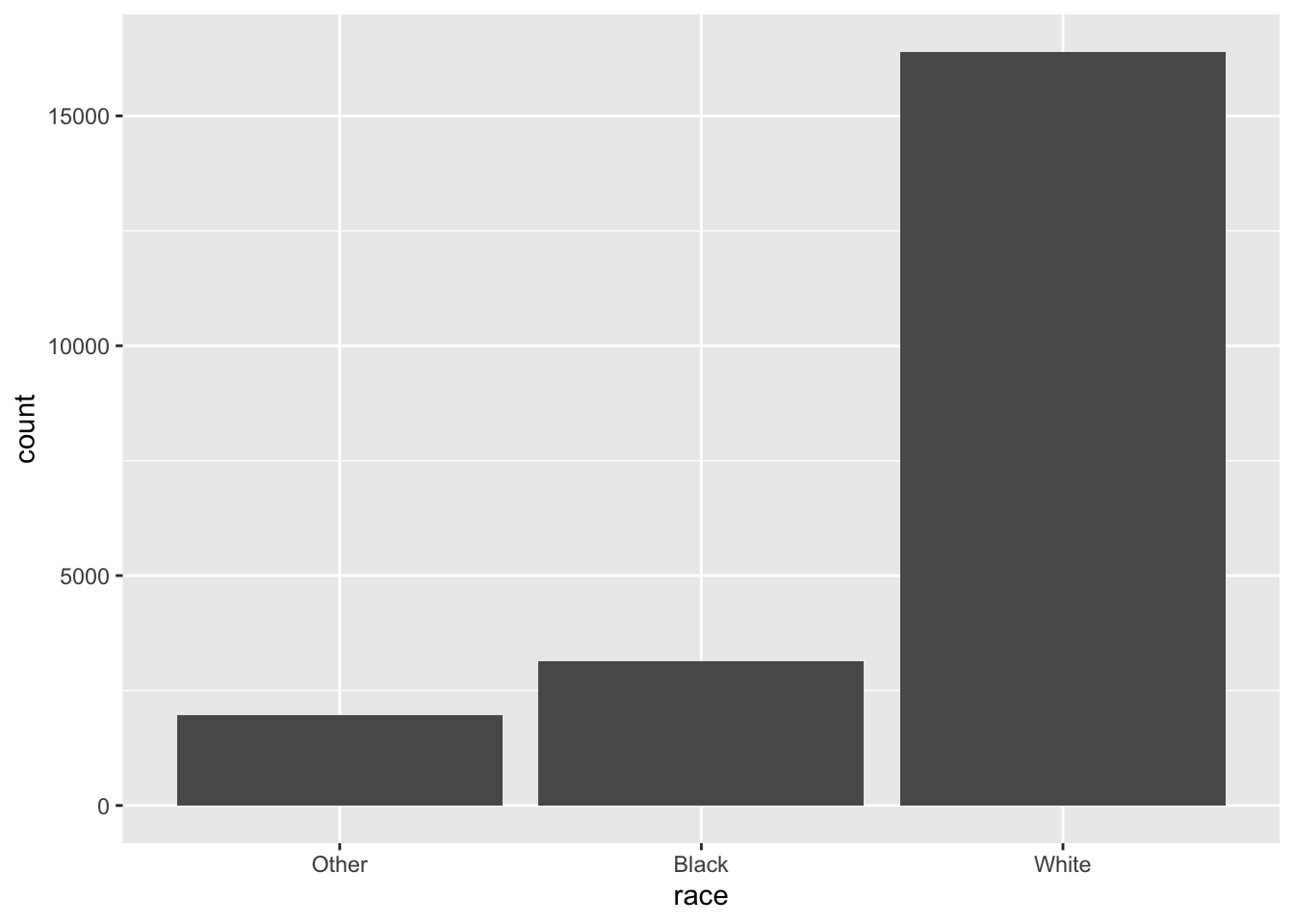Important

When working with factors, the two most common operations are

1. Changing the order of the levels
2. Changing the values of the levels

Those operations are described in the sections below.

## Modifying factor order

It’s often useful to change the order of the factor levels in a visualization.

Let’s explore the `relig` (religion) factor:

``````gss_cat %>%
count(relig)``````
``````# A tibble: 15 × 2
relig                       n
<fct>                   <int>
2 Don't know                 15
3 Inter-nondenominational   109
4 Native american            23
5 Christian                 689
6 Orthodox-christian         95
7 Moslem/islam              104
8 Other eastern              32
9 Hinduism                   71
10 Buddhism                  147
11 Other                     224
12 None                     3523
13 Jewish                    388
14 Catholic                 5124
15 Protestant              10846``````

We see there are 15 categories in the `gss_cat` dataset.

``attributes(gss_cat\$relig)``
``````\$levels
 "Inter-nondenominational" "Native american"
 "Christian"               "Orthodox-christian"
 "Moslem/islam"            "Other eastern"
 "Hinduism"                "Buddhism"
 "Other"                   "None"
 "Jewish"                  "Catholic"
 "Protestant"              "Not applicable"

\$class
 "factor"``````

The first level is “No answer” followed by “Don’t know”, and so on.

Imagine you want to explore the average number of hours spent watching TV (`tvhours`) per day across religions (`relig`):

``````relig_summary <- gss_cat %>%
group_by(relig) %>%
summarise(tvhours = mean(tvhours, na.rm = TRUE),
n = n())

relig_summary %>%
ggplot(aes(x = tvhours, y = relig)) +
geom_point()``````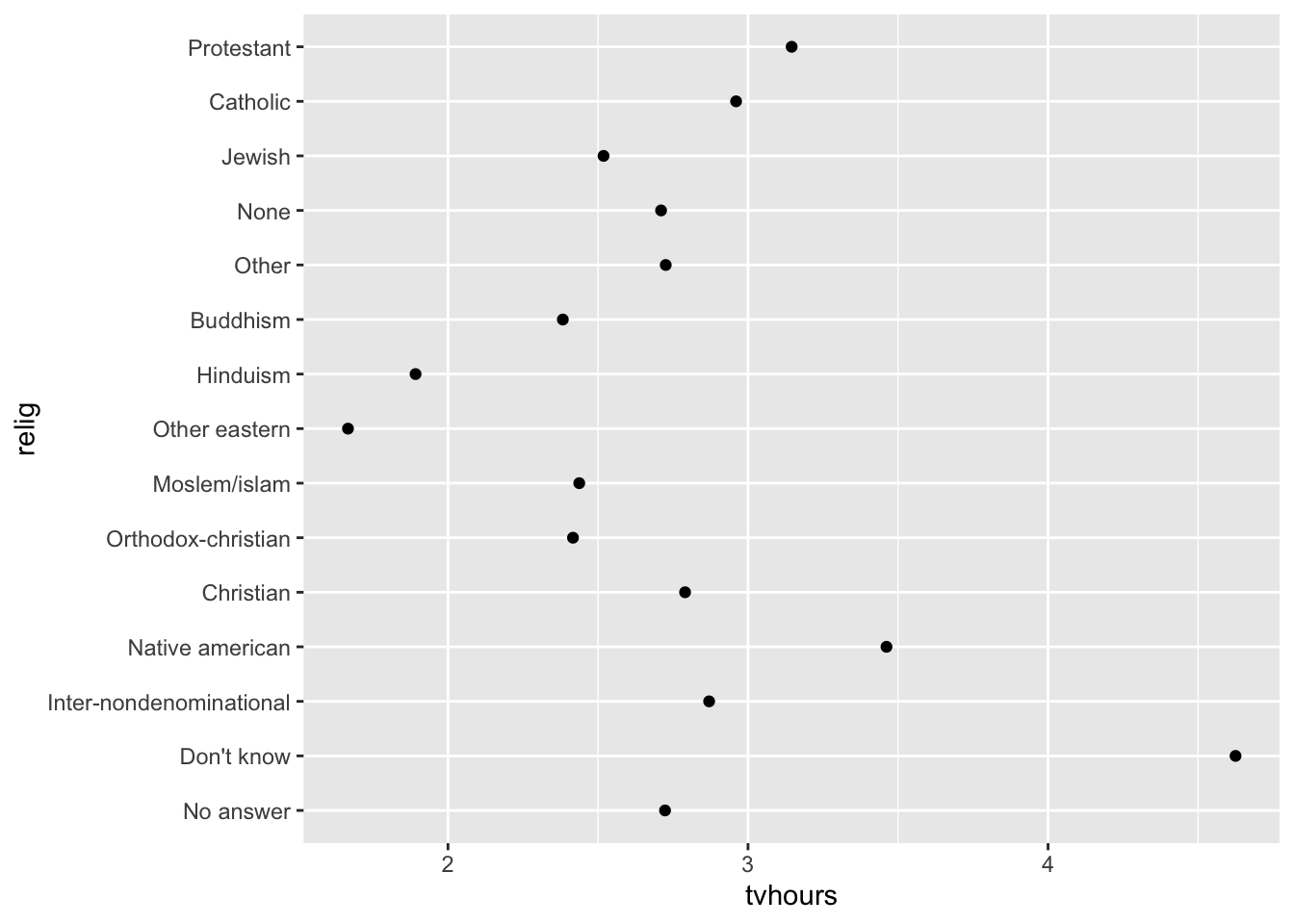The y-axis lists the levels of the `relig` factor in the order of the levels.

However, it is hard to read this plot because there’s no overall pattern.

### `fct_reorder`

We can improve it by reordering the levels of `relig` using `fct_reorder()`. `fct_reorder(.f, .x, .fun)` takes three arguments:

• `.f`, the factor whose levels you want to modify.
• `.x`, a numeric vector that you want to use to reorder the levels.
• Optionally, `.fun`, a function that’s used if there are multiple values of `x` for each value of `f`. The default value is `median`.
``````relig_summary %>%
ggplot(aes(x = tvhours,
y = fct_reorder(.f = relig, .x = tvhours))) +
geom_point()``````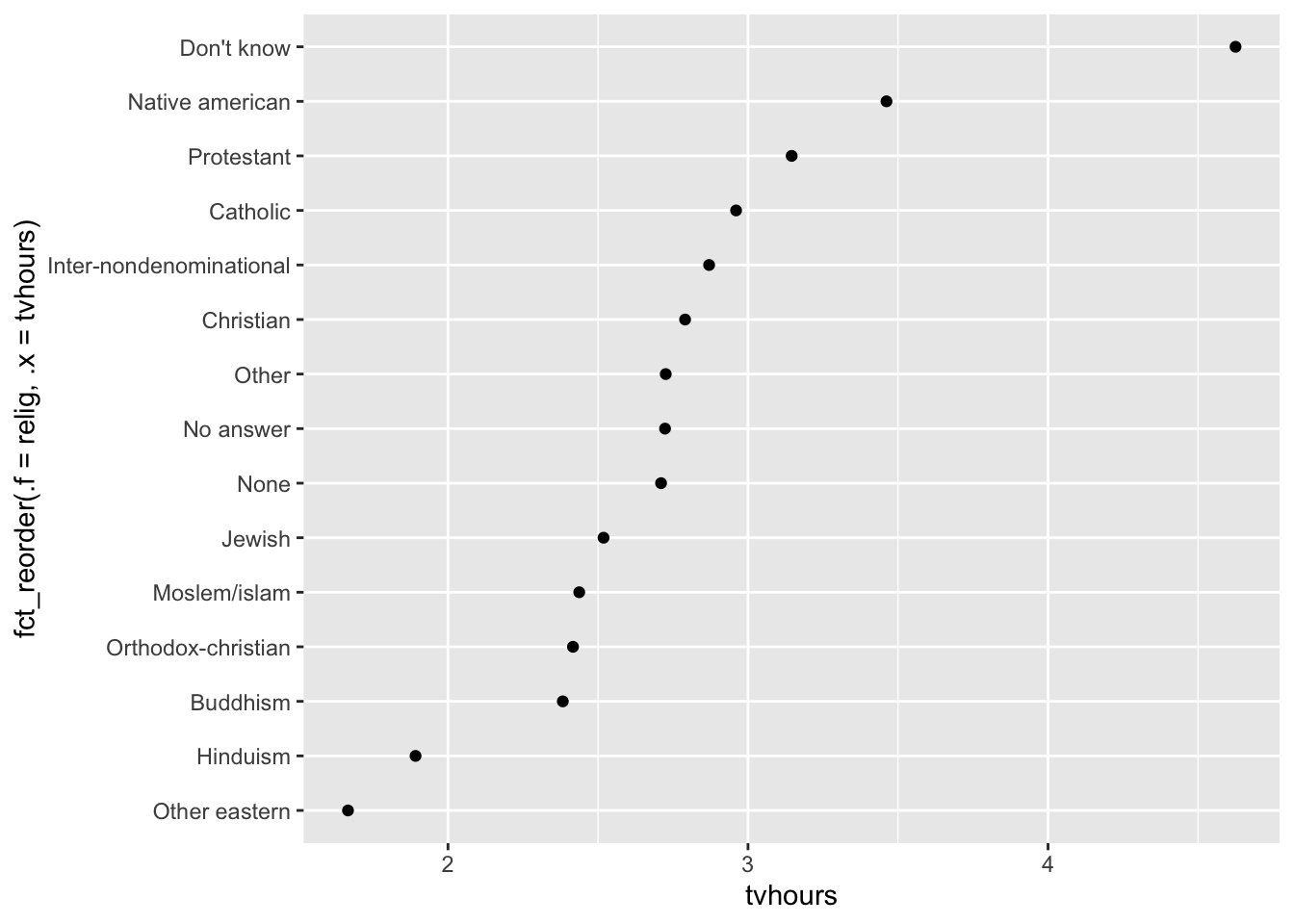Reordering religion makes it much easier to see that people in the “Don’t know” category watch much more TV, and Hinduism & Other Eastern religions watch much less.

As you start making more complicated transformations, I recommend moving them out of `aes()` and into a separate `mutate()` step.

Example

You could rewrite the plot above as:

``````relig_summary %>%
mutate(relig = fct_reorder(relig, tvhours)) %>%
ggplot(aes(x = tvhours, y = relig)) +
geom_point()``````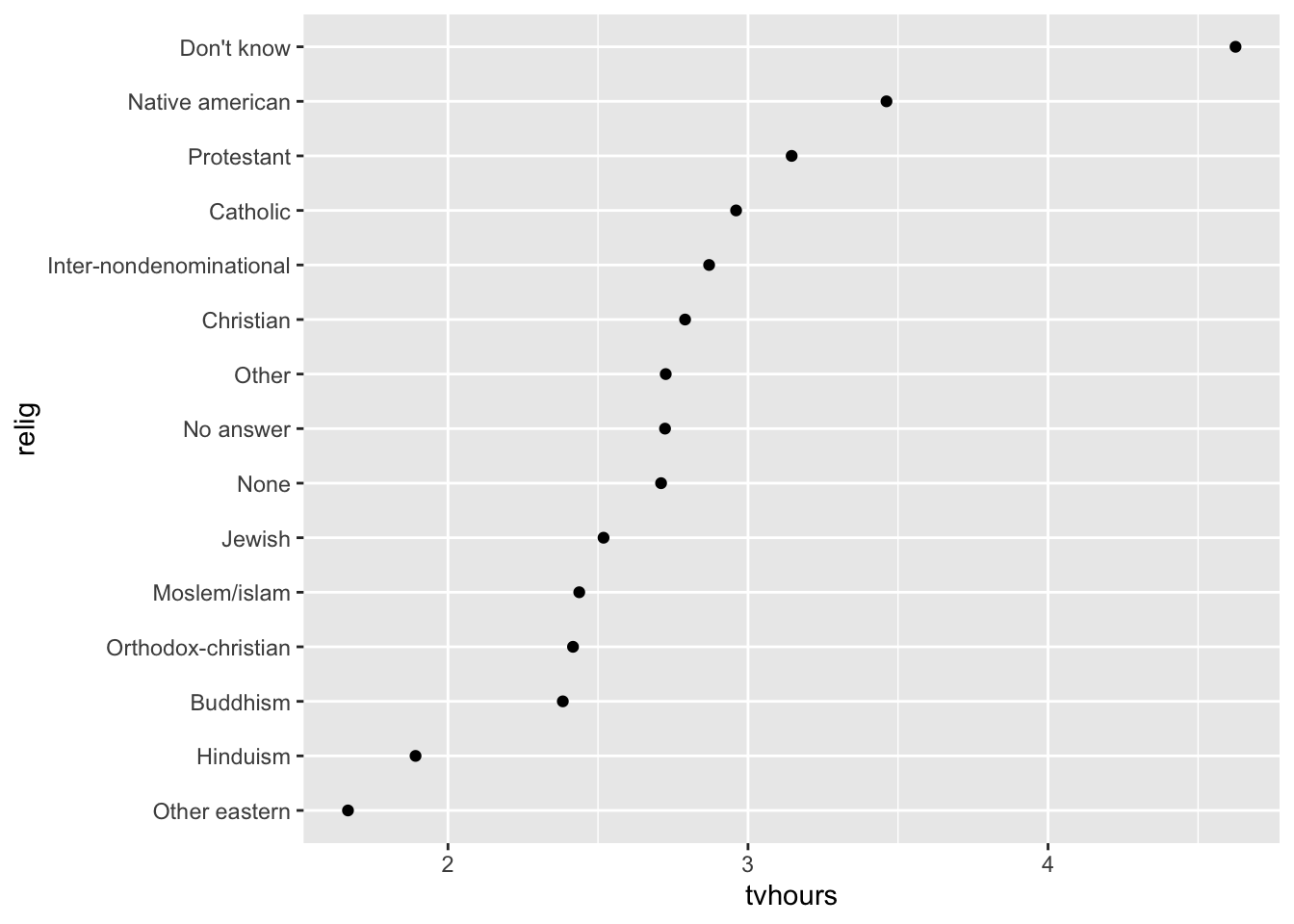Another example

What if we create a similar plot looking at how average age varies across reported income level?

``````rincome_summary <-
gss_cat %>%
group_by(rincome) %>%
summarise(age = mean(age, na.rm = TRUE),
n = n())

rincome_summary %>%
ggplot(aes(x = age, y = fct_reorder(.f = rincome, .x = age))) +
geom_point()``````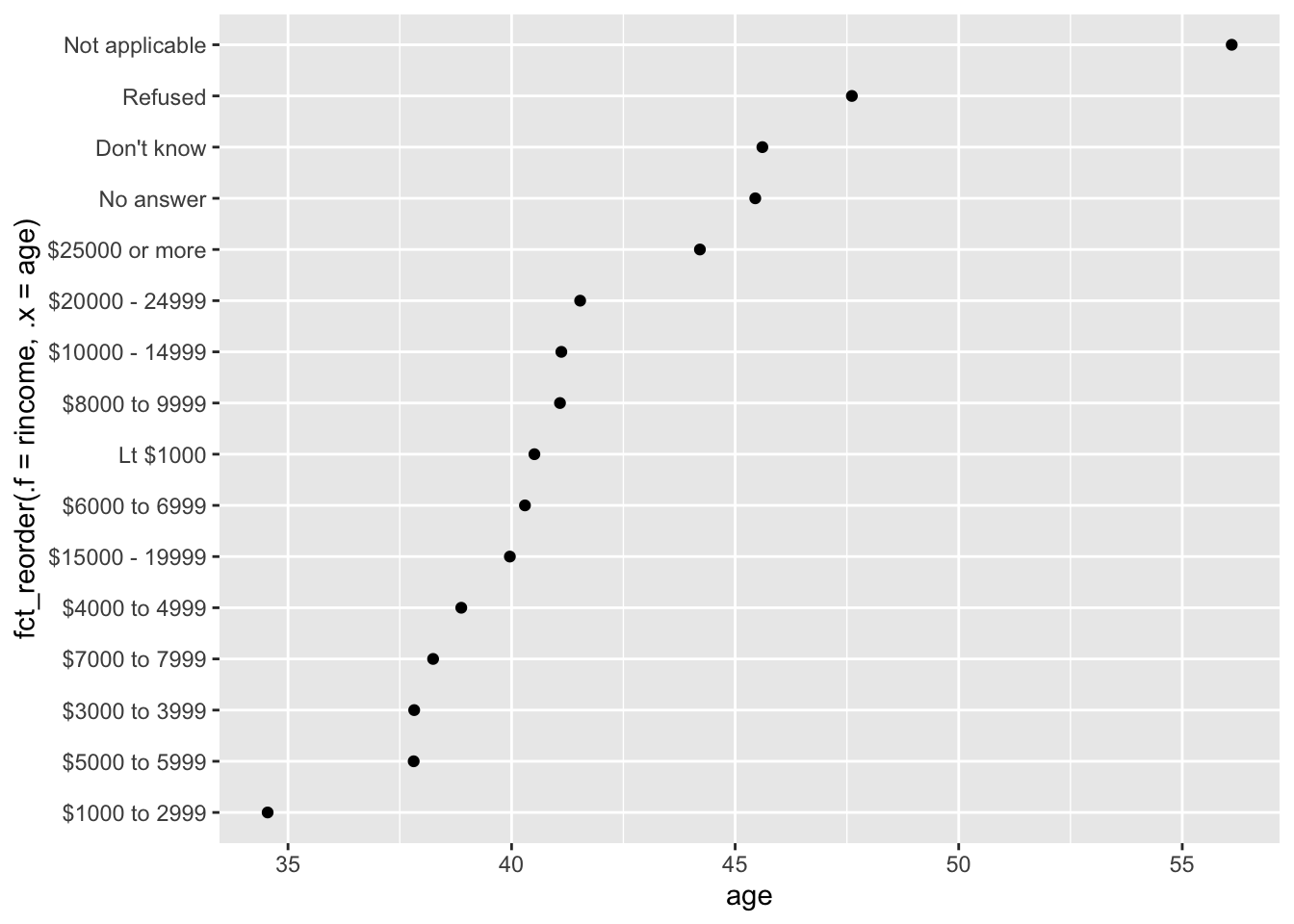Here, arbitrarily reordering the levels isn’t a good idea! That’s because `rincome` already has a principled order that we shouldn’t mess with.

Pro-tip

Reserve `fct_reorder()` for factors whose levels are arbitrarily ordered.

Question

Let’s practice `fct_reorder()`. Using the `palmerpenguins` dataset,

1. Calculate the average `bill_length_mm` for each species
2. Create a scatter plot showing the average for each species.
3. Go back and reorder the factor `species` based on the average bill length from largest to smallest.
4. Now order it from smallest to largest
``````library(palmerpenguins)
penguins ``````
``````# A tibble: 344 × 8
species island    bill_length_mm bill_depth_mm flipper_…¹ body_…² sex    year
<fct>   <fct>              <dbl>         <dbl>      <int>   <int> <fct> <int>
1 Adelie  Torgersen           39.1          18.7        181    3750 male   2007
2 Adelie  Torgersen           39.5          17.4        186    3800 fema…  2007
3 Adelie  Torgersen           40.3          18          195    3250 fema…  2007
4 Adelie  Torgersen           NA            NA           NA      NA <NA>   2007
5 Adelie  Torgersen           36.7          19.3        193    3450 fema…  2007
6 Adelie  Torgersen           39.3          20.6        190    3650 male   2007
7 Adelie  Torgersen           38.9          17.8        181    3625 fema…  2007
8 Adelie  Torgersen           39.2          19.6        195    4675 male   2007
9 Adelie  Torgersen           34.1          18.1        193    3475 <NA>   2007
10 Adelie  Torgersen           42            20.2        190    4250 <NA>   2007
# … with 334 more rows, and abbreviated variable names ¹​flipper_length_mm,
#   ²​body_mass_g``````
``## Try it out``

### `fct_relevel`

However, it does make sense to pull “Not applicable” to the front with the other special levels.

You can use `fct_relevel()`.

It takes a factor, `f`, and then any number of levels that you want to move to the front of the line.

``````rincome_summary %>%
ggplot(aes(age, fct_relevel(rincome, "Not applicable"))) +
geom_point()``````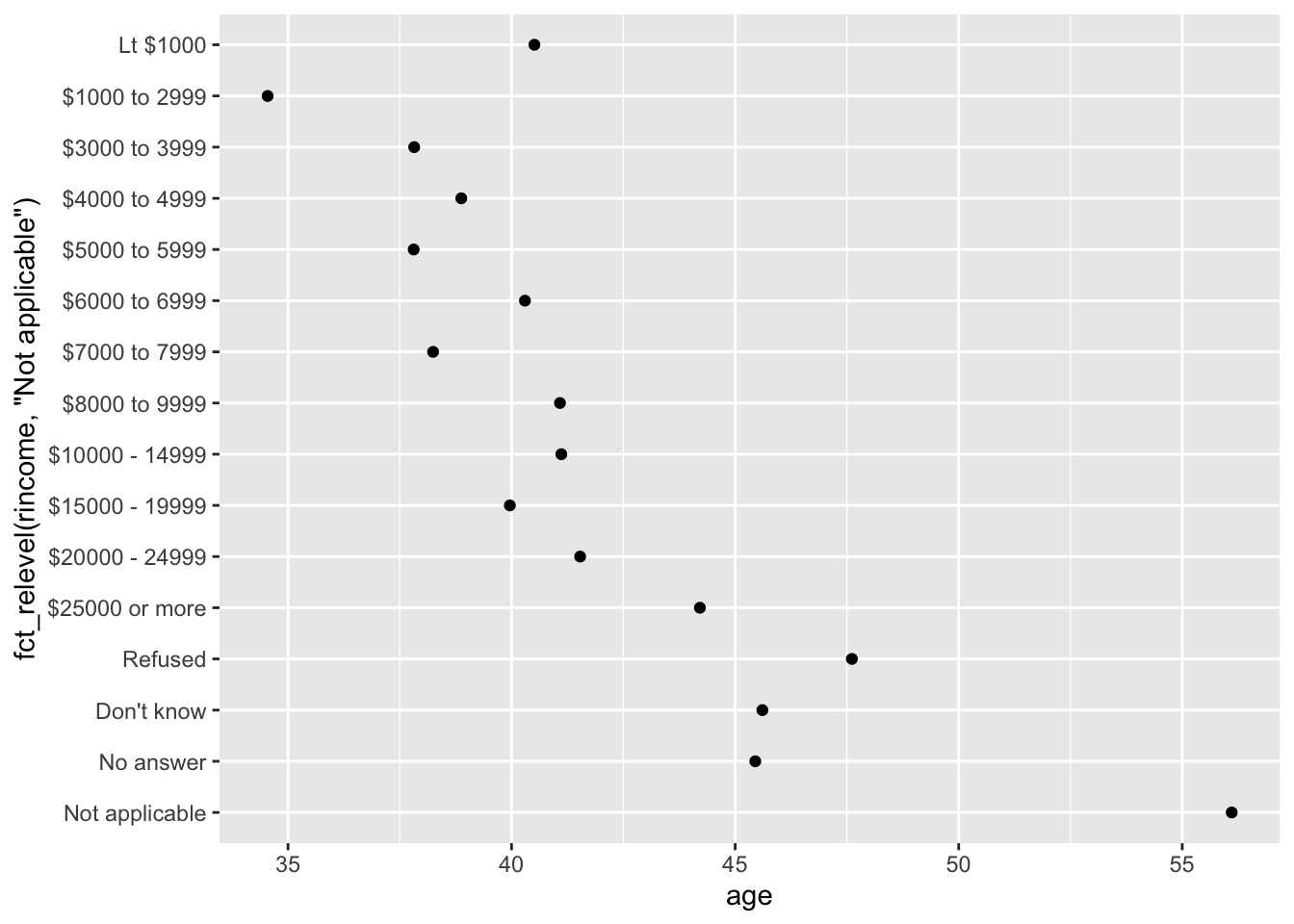Note

Any levels not mentioned in `fct_relevel` will be left in their existing order.

Another type of reordering is useful when you are coloring the lines on a plot. `fct_reorder2(f, x, y)` reorders the factor `f` by the `y` values associated with the largest `x` values.

This makes the plot easier to read because the colors of the line at the far right of the plot will line up with the legend.

``````by_age <-
gss_cat %>%
filter(!is.na(age)) %>%
count(age, marital) %>%
group_by(age) %>%
mutate(prop = n / sum(n))

by_age %>%
ggplot(aes(age, prop, colour = marital)) +
geom_line(na.rm = TRUE)
by_age %>%
ggplot(aes(age, prop, colour = fct_reorder2(marital, age, prop))) +
geom_line() +
labs(colour = "marital")``````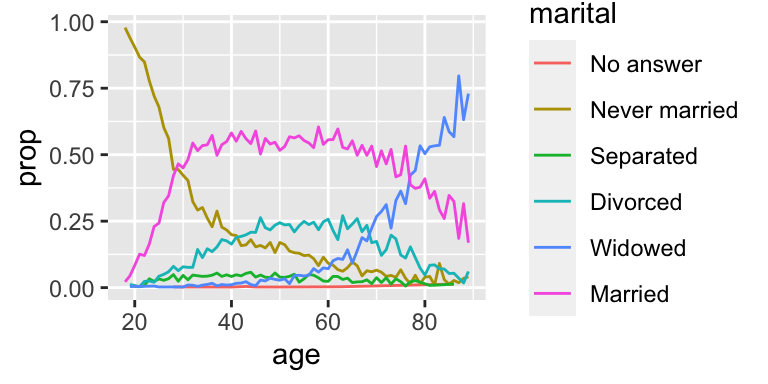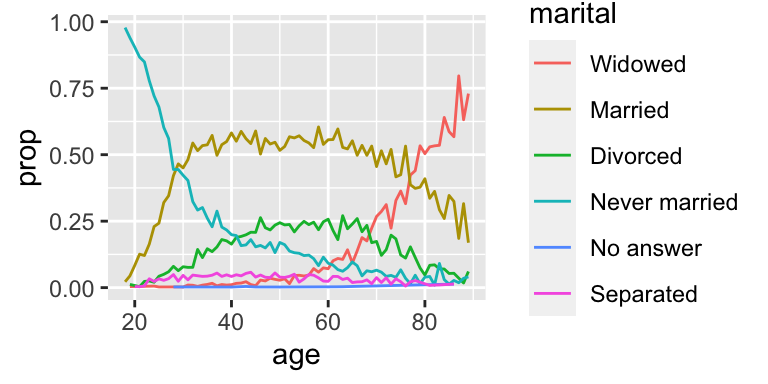### `fct_infreq`

Finally, for bar plots, you can use `fct_infreq()` to order levels in decreasing frequency: this is the simplest type of reordering because it doesn’t need any extra variables. Combine it with `fct_rev()` if you want them in increasing frequency so that in the bar plot largest values are on the right, not the left.

``````gss_cat %>%
mutate(marital = marital %>% fct_infreq() %>% fct_rev()) %>%
ggplot(aes(marital)) +
geom_bar()``````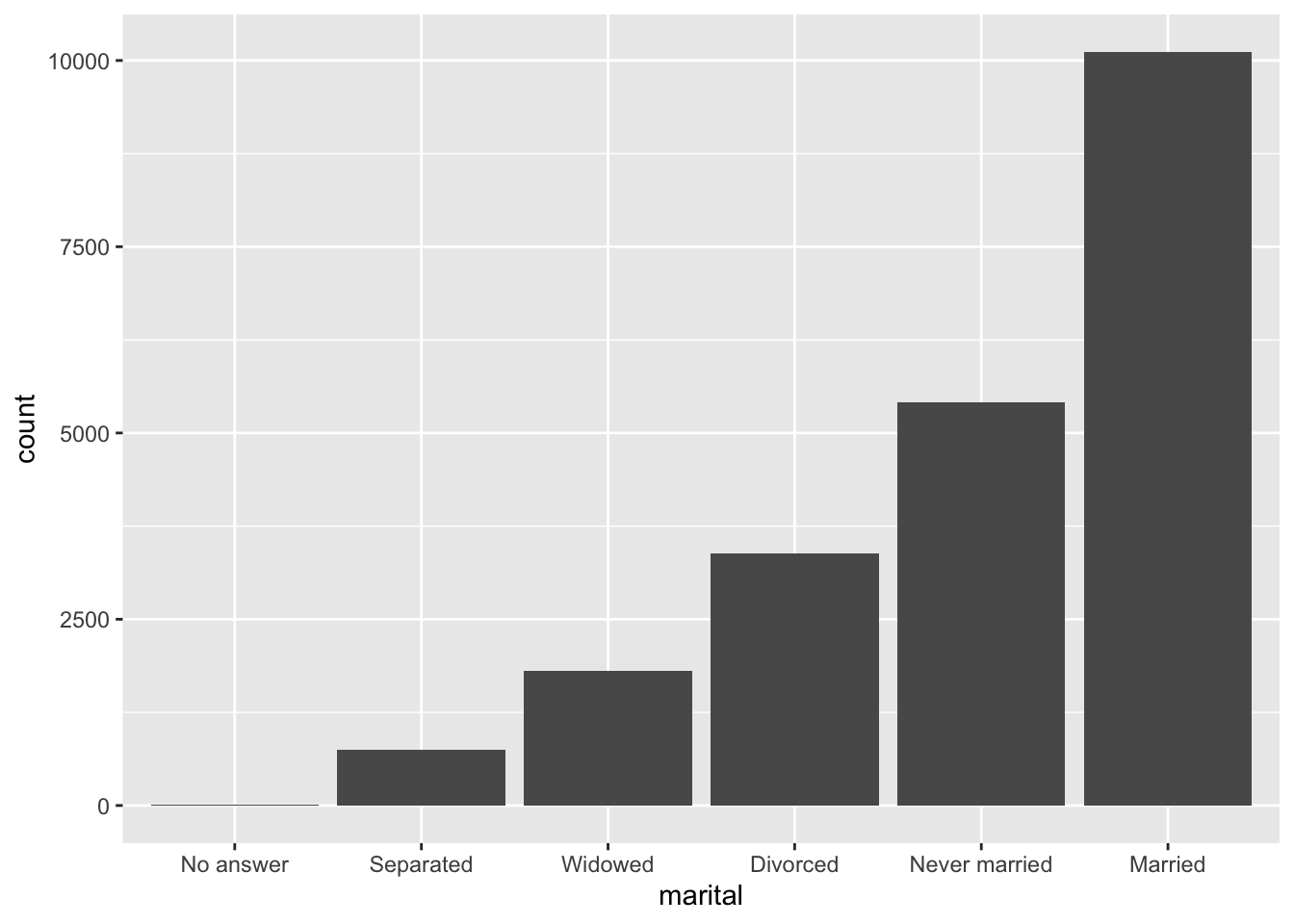## Modifying factor levels

More powerful than changing the orders of the levels is changing their values. This allows you to clarify labels for publication, and collapse levels for high-level displays.

### `fct_recode`

The most general and powerful tool is `fct_recode()`. It allows you to recode, or change, the value of each level. For example, take the `gss_cat\$partyid`:

``````gss_cat %>%
count(partyid)``````
``````# A tibble: 10 × 2
partyid                n
<fct>              <int>
2 Don't know             1
3 Other party          393
4 Strong republican   2314
5 Not str republican  3032
6 Ind,near rep        1791
7 Independent         4119
8 Ind,near dem        2499
9 Not str democrat    3690
10 Strong democrat     3490``````

The levels are terse and inconsistent.

Let’s tweak them to be longer and use a parallel construction.

Like most rename and recoding functions in the tidyverse:

• the new values go on the left
• the old values go on the right
``````gss_cat %>%
mutate(partyid = fct_recode(partyid,
"Republican, strong"    = "Strong republican",
"Republican, weak"      = "Not str republican",
"Independent, near rep" = "Ind,near rep",
"Independent, near dem" = "Ind,near dem",
"Democrat, weak"        = "Not str democrat",
"Democrat, strong"      = "Strong democrat")) %>%
count(partyid)``````
``````# A tibble: 10 × 2
partyid                   n
<fct>                 <int>
2 Don't know                1
3 Other party             393
4 Republican, strong     2314
5 Republican, weak       3032
6 Independent, near rep  1791
7 Independent            4119
8 Independent, near dem  2499
9 Democrat, weak         3690
10 Democrat, strong       3490``````
Note

`fct_recode()` will leave the levels that aren’t explicitly mentioned as is, and will warn you if you accidentally refer to a level that doesn’t exist.

To combine groups, you can assign multiple old levels to the same new level:

``````gss_cat %>%
mutate(partyid = fct_recode(partyid,
"Republican, strong"    = "Strong republican",
"Republican, weak"      = "Not str republican",
"Independent, near rep" = "Ind,near rep",
"Independent, near dem" = "Ind,near dem",
"Democrat, weak"        = "Not str democrat",
"Democrat, strong"      = "Strong democrat",
"Other"                 = "Don't know",
"Other"                 = "Other party")) %>%
count(partyid)``````
``````# A tibble: 8 × 2
partyid                   n
<fct>                 <int>
1 Other                   548
2 Republican, strong     2314
3 Republican, weak       3032
4 Independent, near rep  1791
5 Independent            4119
6 Independent, near dem  2499
7 Democrat, weak         3690
8 Democrat, strong       3490``````

Use this technique with care: if you group together categories that are truly different you will end up with misleading results.

### `fct_collapse`

If you want to collapse a lot of levels, `fct_collapse()` is a useful variant of `fct_recode()`.

For each new variable, you can provide a vector of old levels:

``````gss_cat %>%
mutate(partyid = fct_collapse(partyid,
"other" = c("No answer", "Don't know", "Other party"),
"rep" = c("Strong republican", "Not str republican"),
"ind" = c("Ind,near rep", "Independent", "Ind,near dem"),
"dem" = c("Not str democrat", "Strong democrat"))) %>%
count(partyid)``````
``````# A tibble: 4 × 2
partyid     n
<fct>   <int>
1 other     548
2 rep      5346
3 ind      8409
4 dem      7180``````

### `fct_lump_*`

Sometimes you just want to lump together the small groups to make a plot or table simpler.

That’s the job of the `fct_lump_*()` family of functions.

`fct_lump_lowfreq()` is a simple starting point that progressively lumps the smallest groups categories into “Other”, always keeping “Other” as the smallest category.

``````gss_cat %>%
mutate(relig = fct_lump_lowfreq(relig)) %>%
count(relig)``````
``````# A tibble: 2 × 2
relig          n
<fct>      <int>
1 Protestant 10846
2 Other      10637``````

In this case it’s not very helpful: it is true that the majority of Americans in this survey are Protestant, but we’d probably like to see some more details!

Instead, we can use the `fct_lump_n()` to specify that we want exactly 10 groups:

``````gss_cat %>%
mutate(relig = fct_lump_n(relig, n = 10)) %>%
count(relig, sort = TRUE) %>%
print(n = Inf)``````
``````# A tibble: 10 × 2
relig                       n
<fct>                   <int>
1 Protestant              10846
2 Catholic                 5124
3 None                     3523
4 Christian                 689
5 Other                     458
6 Jewish                    388
7 Buddhism                  147
8 Inter-nondenominational   109
9 Moslem/islam              104
10 Orthodox-christian         95``````

Read the documentation to learn about `fct_lump_min()` and `fct_lump_prop()` which are useful in other cases.

## Ordered factors

There’s a special type of factor that needs to be mentioned briefly: ordered factors.

Ordered factors, created with `ordered()`, imply a strict ordering and equal distance between levels:

The first level is “less than” the second level by the same amount that the second level is “less than” the third level, and so on…

You can recognize them when printing because they use `<` between the factor levels:

``ordered(c("a", "b", "c"))``
`````` a b c
Levels: a < b < c``````

However, in practice, `ordered()` factors behave very similarly to regular factors.

# Post-lecture materials

### Final Questions

Questions
1. Explore the distribution of `rincome` (reported income). What makes the default bar chart hard to understand? How could you improve the plot?

2. What is the most common `relig` in this survey? What’s the most common `partyid`?

3. Which `relig` does `denom` (denomination) apply to? How can you find out with a table? How can you find out with a visualization?

4. There are some suspiciously high numbers in `tvhours`. Is the mean a good summary?

5. For each factor in `gss_cat` identify whether the order of the levels is arbitrary or principled.

6. Why did moving “Not applicable” to the front of the levels move it to the bottom of the plot?

7. How have the proportions of people identifying as Democrat, Republican, and Independent changed over time?

8. How could you collapse `rincome` into a small set of categories?

9. Notice there are 9 groups (excluding other) in the `fct_lump` example above. Why not 10? (Hint: type `?fct_lump`, and find the default for the argument `other_level` is “Other”.)